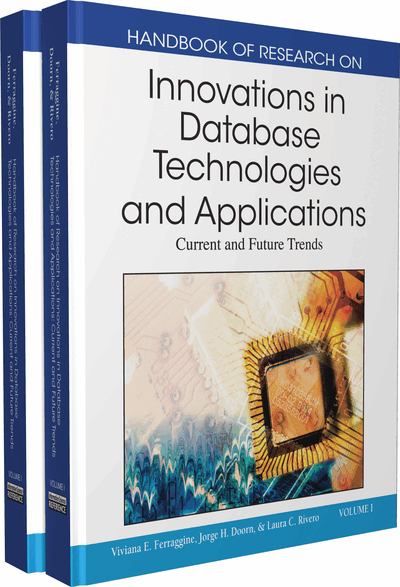# Mining Frequent Closed Itemsets for Association Rules

Anamika Gupta (University of Delhi, India), Shikha Gupta (University of Delhi, India) and Naveen Kumar (University of Delhi, India)
DOI: 10.4018/978-1-60566-242-8.ch057
Available
\$37.50
No Current Special Offers

## Abstract

Association refers to correlations that exist among data. Association Rule Mining (ARM) is an important data-mining task. It refers to discovery of rules between different sets of attributes/items in very large databases (Agrawal R. & Srikant R. 1994). The discovered rules help in strategic decision making in both commercial and scientific domains. A classical application of ARM is market basket analysis, an application of data mining in retail sales where associations between the different items are discovered to analyze the customer’s buying habits in order to develop better marketing strategies. ARM has been extensively used in other applications like spatial-temporal, health care, bioinformatics, web data etc (Han J., Cheng H., Xin D., & Yan X. 2007).
Chapter Preview
Top

## Background

Let D denotes the database of N transactions and I denotes the set of n items in D. A set of one or more items belonging to set I is termed as an itemset. A k-itemset is an itemset of cardinality k. A transaction TD contains an itemset and is associated with a unique identifier TID. The probability of an itemset X being contained in a transaction is termed as support of X.

An itemset having support greater than the user specified support threshold (ms) is termed as frequent itemset (FI).

An association rule is an implication of the form X →Y where XI, YI and XY =∅. Support and Confidence are rule evaluation metrics of an association rule. Support of a rule X → Y in D is ‘sup’ if sup% of transactions in D contain XY. It is computed as:

Confidence of a rule X → Y in D is ‘conf’ if conf% of transactions in D that contain X, also contain Y. It is computed, as the conditional probability that Y occurs in a transaction given X is present in the same transaction, i.e.

A rule generated from frequent itemsets is strong if its confidence is greater than the user specified confidence threshold (mc).

Three independent groups of researchers (Pasquier N., Bastide Y., Taouil R. & Lakhal L. 1999a), (Zaki M.J. & Hsiao C. J. 1999), (Stumme G., 1999) introduced the notion of mining FCI instead of FI and have given the following definitions of FCI:

Zaki et al. defines closed itemset as an itemset whose support is not equal to support of any of its proper superset (Closure Property) (Zaki M. J. & Hsiao C. J. 1999). In other words X is a closed itemset if there exists no proper superset X’ of X such that support(X) = support(X’). Closed itemsets with support greater than the user specified support threshold (ms) are frequent closed itemsets (FCI).

## Key Terms in this Chapter

Data Mining: Extraction of interesting, non-trivial, implicit, previously unknown and potentially useful information or patterns from data in large databases

Formal Concept Analysis (FCA): It is a branch of mathematics based on Concept and Concept Hierarchies.

Galois Closure Operator: Let D = (O,I,R) be a data mining context where O and I are finite sets of objects (transactions) and items respectively. ? ? ? x ? is a binary relation between objects and items. For O ? O, and I ? I, we define: f(O): 2O ? 2If(O) = (i? I | ?o ? O, (o,i) ? R} g(I): 2I ? 2Og(I) = (o? O | ?i ? I, (o,i) ? R} f(O) associates with O the items common to all objects o ? O and g(I) associates with I the objects related to all items i ? I. The operators h = f o g in 2I and h’ = g o f in 2O are Galois closure operators. An itemset C ? I from D is a closed itemset iff h(C) = C.

Association Rule: An Association rule is an implication of the form X?Y where X ? I, Y? I and XnY =Ø, I denotes the set of items.

Formal Concept: A formal context K = (G,M,I) consists of two sets G (objects) and M (attributes) and a relation I between G and M. For a set A?G of objects A’={meM | gIm for all geA} (the set of all attributes common to the objects in A). Correspondingly, for a set B of attributes we define B’ = {geG | gIm for all meB} (the set of objects common to the attributes in B). A formal concept of the context (G,M,I) is a pair (A,B) with A?G,B?M, such that A’=B and B’=A A is called the extent and B is the intent of the Formal Concept (A,B).

Closure: For any itemset C ? I, h(C) is the closure of C where h = f o g is the Galois Closure Operator.

Frequent Itemset (FI): A set of one or more items belonging to set I is termed as an itemset. An itemset X is a frequent itemset if support of X is greater than the user specified support threshold (ms).

Non-Redundant Association Rules: Let Ri denote the rule X1i?X2i, where X1,X2 ? I. Rule R1 is more general than rule R2 provided R2 can be generated by adding additional items to either the antecedent or consequent of R1. Rules having the same support and confidence as more general rules are the redundant association rules. Remaining rules are non-redundant rules.

Generator Itemset: A generator p of a closed itemset c is one of the smallest itemsets such that h(p) = c.

Frequent Closed Itemset (FCI): An itemset X is a closed itemset if there exists no itemset X’ such that i. X’ is a proper superset of X, ii. Every transaction containing X also contains X’. A closed itemset X is frequent if its support exceeds the given support threshold.

## Complete Chapter List

Search this Book:
Reset